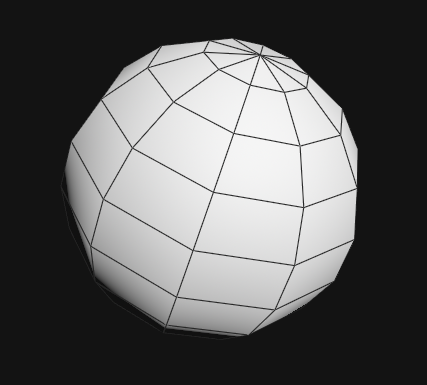Jump to content

## Recommended Posts

hello, I'm trying to mesh a group of point, from an array.

It gives a meshed sphere but with hole, and overlapping points.

I guess I shd use some condition but I'm not really sure how..

int total =40;
int prim;
float r = 200.0;

float   lon    = -(\$PI); //-pi,pi
float   lat     = -(\$PI)/2;//-pi/2, pi/2

float   step_lon = lon/total; //angle sur la longitude
float   step_lat  = lat/total;//angle sur la latitude

int globe[] = {0,0,0};

for (int i=0 ; i<total+1; i++){
for (int j=0; j< total+2; j++){

//polar to cartesian
float x = r * sin(lon) * cos(lat);
float y = r * sin(lon) * sin(lat);
float z = r * cos(lon);

//vector pos
vector pos = set(x,y,z);

//feed array, create points sphere
globe[j]=addpoint(geoself(), pos);

int v1 = globe[j];
int v2 = globe[j+1];
int v3 = globe[j+2];

prim = addprim(geoself(), "poly");

addvertex(geoself(), prim, v1);
addvertex(geoself(), prim, v2);
addvertex(geoself(), prim, v3);

//setpointattrib(geoself(), "lat", v1, j, "set");
// setpointattrib(geoself(), "lat", v2, j+1, "set");
//setpointattrib(geoself(), "lat", v3, j+2, "set");

lat += step_lat;
}

lon +=step_lon;

}

IT Looks like it miss half of the primitives.

thks

HIP FILE :

Edited by ezza

#### Share this post

##### Share on other sites

Are you trying to generate UV sphere? If I understand your goal correctly, using last 4 points is wrong technique here. It will only produce blade-like polygons. You need to determine primitives's point numbers by looking at generated points's numbers and possibly visualizing i and j values.```// Detail wrangle.

#include "voplib.h"

int rows = chi("rows");
int cols = chi("cols");
float radius = ch("radius");

for (int i = 0; i < rows; i++)
{
float step_lon = PI / (rows - 1);
float lon = i * step_lon;

for (int j = 0; j < cols; j++)
{
float step_lat = 2 * PI / cols;
float lat = j * step_lat;

addpoint(0, vop_frompolar(lat, lon, radius));

if (i+1 == rows)
continue;

int prim = addprim(0, "poly");
int v1 = i*cols + j;
int v2 = i*cols + (j+1)%cols;
int v3 = v2 + cols;
int v4 = v1 + cols;
addvertex(0, prim, v1);
addvertex(0, prim, v2);
addvertex(0, prim, v3);
addvertex(0, prim, v4);
}
}```

uv_sphere.hipnc

•3

#### Share this post

##### Share on other sites

I cannot say how much I'm thankfull!! thas exactly what I was looking for, next exercice icosphere!

I may come back for other noob questions!!

thks a lot, great forum!!

## Create an account or sign in to comment

You need to be a member in order to leave a comment

## Create an account

Sign up for a new account in our community. It's easy!

Register a new account

## Sign in

Already have an account? Sign in here.

Sign In Now

×
• Donations

• Leaderboard